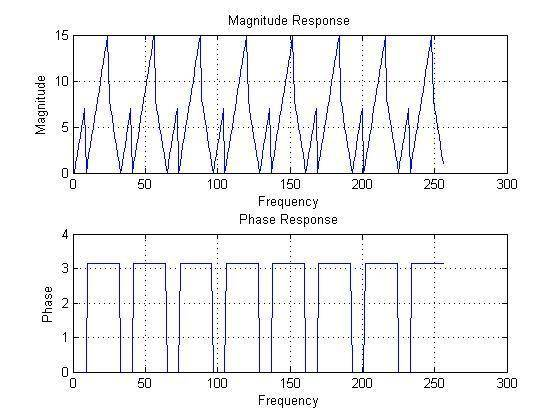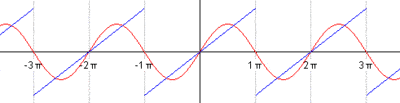# Creating a triangular waveform in Matlab

## Homework Statement

Create 512 element triangular waveform (In Matlab)

x(k)= k 0≤k≤7
8-k 8≤k≤23
-32+k 24≤k≤31

For 0≤k≤31

repeating every 32 elements.

N/A

## The Attempt at a Solution

Ok so im confused on how to code this.

This is what I have so far.

k1=0:7;
k2=8:23;
k3=8-k2;
k4=24:31;
k5=-32+k4;
K=[k1,k3,k5];

Im not sure if this is the best way though. Or if this is correct.

How do I get it to repeat every 32 elements?

Thanks for any assistance!

## The Attempt at a Solution

Simon Bridge
Homework Helper
You can range-index inside vectors eg.

k=0:31;
z = [k(1:8) 8-k(9:24) k(25:32)-32];

This assignment is an indexing exercize - since z is 32 elements long, and you want x to be 512 elements long - repeating z, then you could just do:

x=[z z z z z z z z z z z z z z z z];

which is pretty apt.
You will be expected to look for some indexing method perhaps like

x(1:16)=z;

only that won't work - go through your notes for the method to stick a vector in an indexed position.

Last edited:
Hi Simon!

Thanks for the info. This makes the code much cleaner. Plus if I want to change something it will be much easier to implement.

After creating this array i am trying to attempt to calculate the fft and plot the magnitude and phase.

I have done this (I think)

Here is my code:
k=0:31;
Z=[k(1:8) 8-k(9:24) k(25:32)-32];
X=[Z Z Z Z Z Z Z Z Z Z Z Z Z Z Z Z];
fft(X);
magX=abs(X);
phaX= angle(X);
magX=magX(1:256);
phaX=phaX(1:256);
subplot(2,1,1); plot(magX); grid
xlabel('Frequency'); ylabel('Magnitude');
title('Magnitude Response');
subplot(2,1,2); plot(phaX); gridI think this is correct. However Im not really sure what im looking at. Can anyone give me a little bit of an idea of what to look for when analyzing a plot of an FFT magnitude and phase response?

Thanks,

#### Attachments

I like Serena
Homework Helper
In one measurement interval you have 256 samples.

You have a wave with 8 periods of 32 samples each in that interval.

A wave with 8 periods means that you can expect a spike in the frequency magnitude at a frequency of 256/8=32 and there is.
Typically you also get spikes at multiples of this frequency.

In your phase diagrams you see jumps up and down of pi.
I believe in your other thread we found that such a jump is not relevant.

Hi I Like Serena,

I see the 8 larger spikes I assume since I've truncated the data to 256 that these are the 8 periods showing up? Are the smaller spike the multiples at lower amplitudes?

As for the phase plot I'm not sure What other thread you are referring too. Why are these not relevant?

Have I been sleep posting? :)

I like Serena
Homework Helper
Check this post of yours: https://www.physicsforums.com/showpost.php?p=3701217&postcount=10

Typically a wave is constructed of a sine and a number of sines with a multiple of the frequency as you can see here:Furthermore, since your wave fits exactly in your measurement interval it wraps around causing multiples of your frequency and dividers of your frequency.

If your wave does not fit exactly in your measurement interval it looks quite different.
more like this:
http://www.facstaff.bucknell.edu/mastascu/elessonshtml/Freq/Freq4Note8MatlabExample.htm

Thanks for the links and information ILS and Simon!